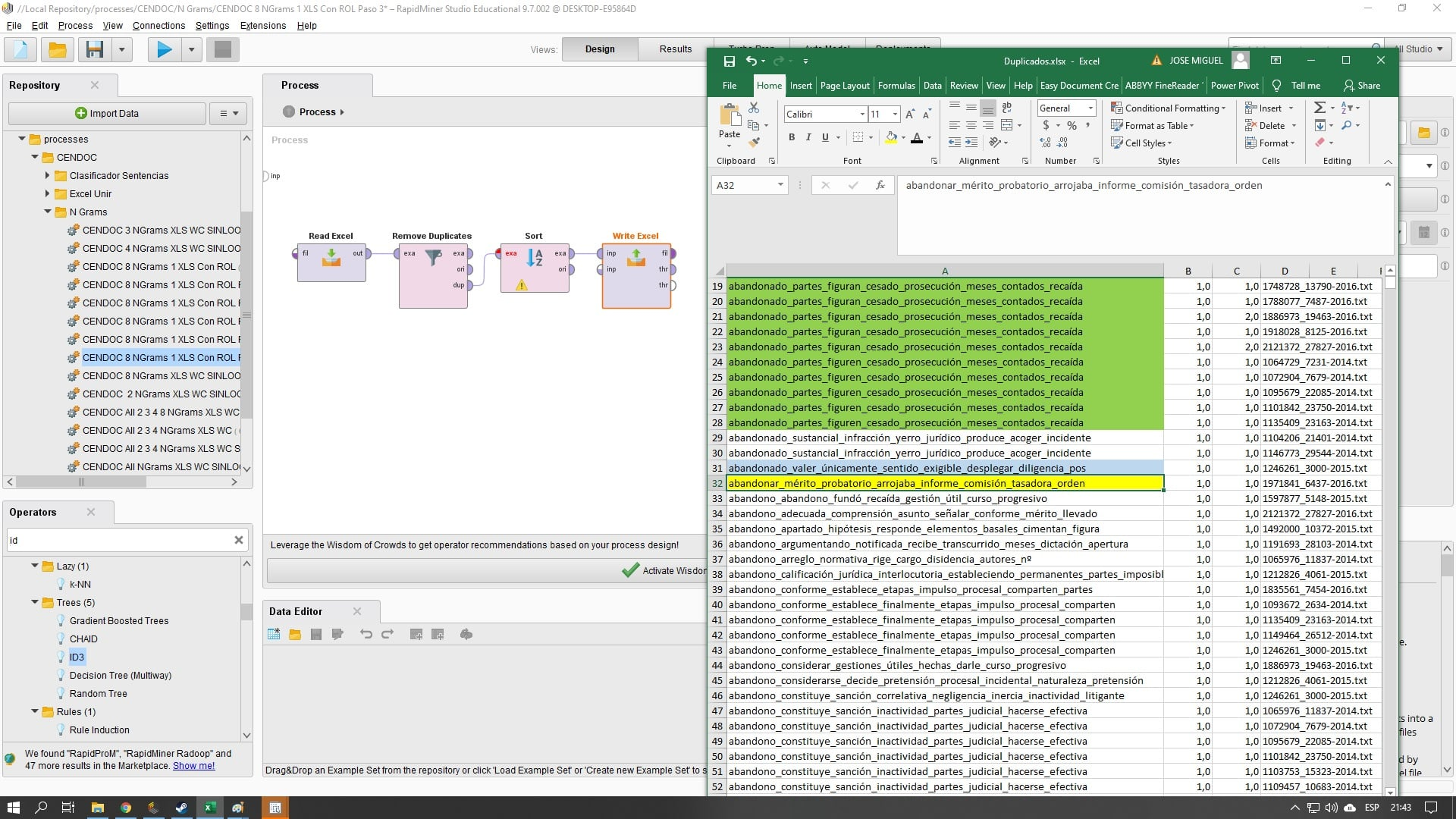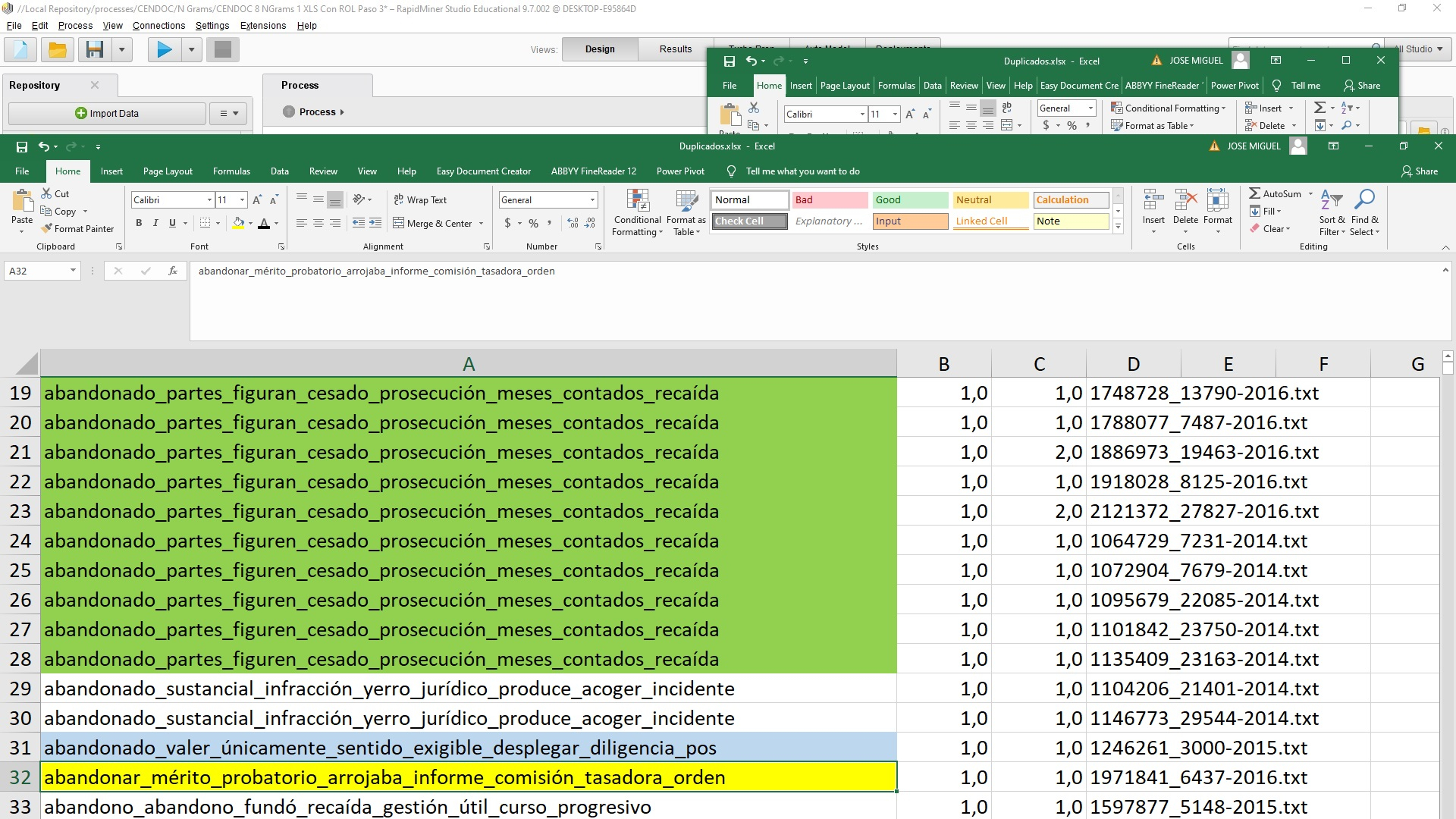# Help!!!!! Remove Duplicates

Member Posts: 18Contributor II
edited August 2020 in Help
Hello: The problem is that after using the remove duplicates operator, when writing those duplicates to an excel spreadsheet, lines that contain results that are not completely duplicates appear, since only the first word matches (Light blue mark)  and some other cases that are not duplicates (yellow mark), Why it could be ?

What I need is that the match is with respect to the 8 words of each line so that they are considered as duplicates and not 1 or 2 or 3 words that match.Tagged:

• Member Posts: 18Contributor II• Administrator, Employee, RapidMiner Certified Analyst, RapidMiner Certified Expert, RMResearcher, Member Posts: 164RM Research
Hi @jmphillips

I expect that the duplicate port of the Remove Duplicates operator just contains the additional Examples (the duplicates), not the original ones. So I expect that the yellow and blue examples occurred exactly two times in the input data set. So one example went to the "exa" output port and the other to the "dup" output port. Thats probably true for the green ones as well. So I expect that the green example occured 29 times in the input data set.

To check for the number of occurences for specific values, you can use this little trick:

```<process version="9.7.001">
<context>
<input/>
<output/>
<macros/>
</context>
<operator activated="true" class="process" compatibility="6.0.002" expanded="true" name="Process" origin="GENERATED_TUTORIAL">
<parameter key="logverbosity" value="init"/>
<parameter key="random_seed" value="2001"/>
<parameter key="send_mail" value="never"/>
<parameter key="process_duration_for_mail" value="30"/>
<parameter key="encoding" value="SYSTEM"/>
<process expanded="true">
<operator activated="true" class="retrieve" compatibility="9.7.001" expanded="true" height="68" name="Golf" origin="GENERATED_TUTORIAL" width="90" x="112" y="85">
<parameter key="repository_entry" value="//Samples/data/Golf"/>
</operator>
<operator activated="true" class="operator_toolbox:group_into_collection" compatibility="2.7.000-SNAPSHOT" expanded="true" height="82" name="Group Into Collection" width="90" x="246" y="85">
<parameter key="group_by_attribute" value="Outlook"/>
<parameter key="group_by_attribute (numerical)" value=""/>
<parameter key="sorting_order" value="none"/>
</operator>
<operator activated="true" class="loop_collection" compatibility="9.7.001" expanded="true" height="82" name="Loop Collection" width="90" x="447" y="85">
<parameter key="set_iteration_macro" value="false"/>
<parameter key="macro_name" value="iteration"/>
<parameter key="macro_start_value" value="1"/>
<parameter key="unfold" value="false"/>
<process expanded="true">
<operator activated="true" class="aggregate" compatibility="9.7.001" expanded="true" height="82" name="Aggregate" width="90" x="380" y="34">
<parameter key="use_default_aggregation" value="false"/>
<parameter key="attribute_filter_type" value="all"/>
<parameter key="attribute" value=""/>
<parameter key="attributes" value=""/>
<parameter key="use_except_expression" value="false"/>
<parameter key="value_type" value="attribute_value"/>
<parameter key="use_value_type_exception" value="false"/>
<parameter key="except_value_type" value="time"/>
<parameter key="block_type" value="attribute_block"/>
<parameter key="use_block_type_exception" value="false"/>
<parameter key="except_block_type" value="value_matrix_row_start"/>
<parameter key="invert_selection" value="false"/>
<parameter key="include_special_attributes" value="false"/>
<parameter key="default_aggregation_function" value="average"/>
<list key="aggregation_attributes">
<parameter key="Outlook" value="count"/>
</list>
<parameter key="group_by_attributes" value="Outlook"/>
<parameter key="count_all_combinations" value="false"/>
<parameter key="only_distinct" value="false"/>
<parameter key="ignore_missings" value="true"/>
</operator>
<connect from_port="single" to_op="Aggregate" to_port="example set input"/>
<connect from_op="Aggregate" from_port="example set output" to_port="output 1"/>
<portSpacing port="source_single" spacing="0"/>
<portSpacing port="sink_output 1" spacing="0"/>
<portSpacing port="sink_output 2" spacing="0"/>
</process>
</operator>
<operator activated="true" class="append" compatibility="9.7.001" expanded="true" height="82" name="Append" width="90" x="648" y="85">
<parameter key="datamanagement" value="double_array"/>
<parameter key="data_management" value="auto"/>
<parameter key="merge_type" value="all"/>
</operator>
<connect from_op="Golf" from_port="output" to_op="Group Into Collection" to_port="exa"/>
<connect from_op="Group Into Collection" from_port="col" to_op="Loop Collection" to_port="collection"/>
<connect from_op="Loop Collection" from_port="output 1" to_op="Append" to_port="example set 1"/>
<connect from_op="Append" from_port="merged set" to_port="result 1"/>
<portSpacing port="source_input 1" spacing="0"/>
<portSpacing port="sink_result 1" spacing="90"/>
<portSpacing port="sink_result 2" spacing="0"/>
</process>
</operator>
</process>
```